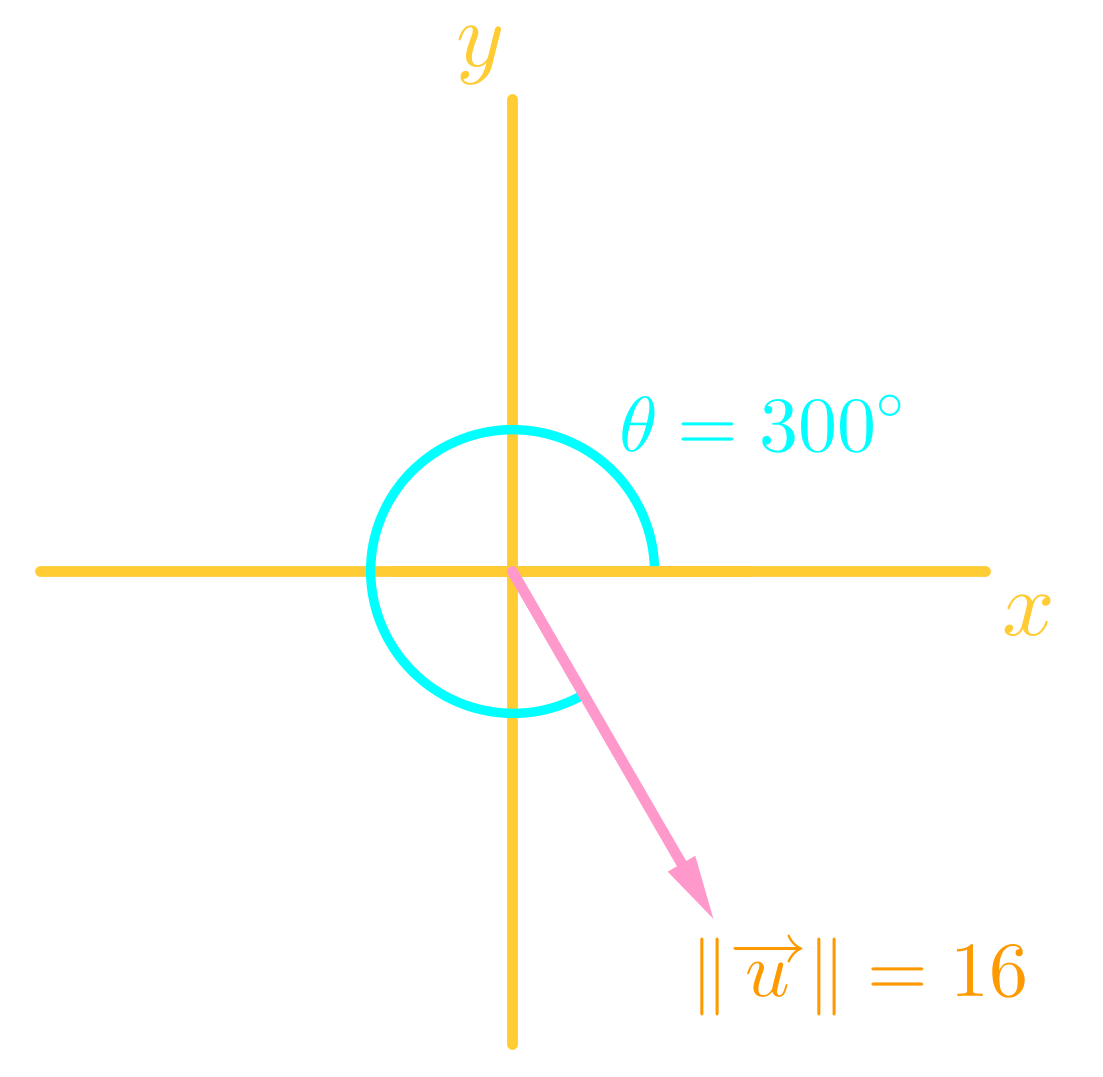# Direction angle of a vector

### Direction angle of a vector

It should be clear by now that a quantity will not be considered a vector quantity if the magnitude or the direction is missing. In this section, we will shift our focus to learn how to indicate the direction of a vector.

#### Lessons

• 1.
Determine the direction angle of the following vectors:
a)
$\vec{p}=$<6, 1>

b)
$\vec{q}=$<-1, 1>

c)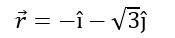d)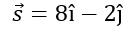• 2.
Given the magnitude and the direction angle of the following vector, determine its component form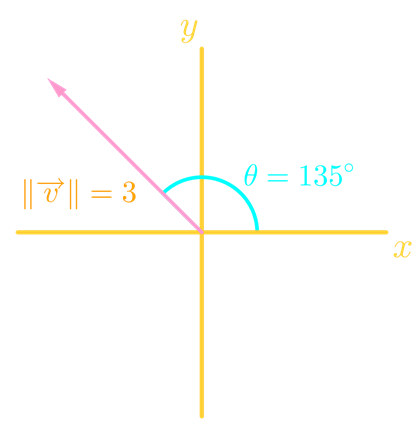• 3.
Given the magnitude and the direction angle of the following vector, determine its component form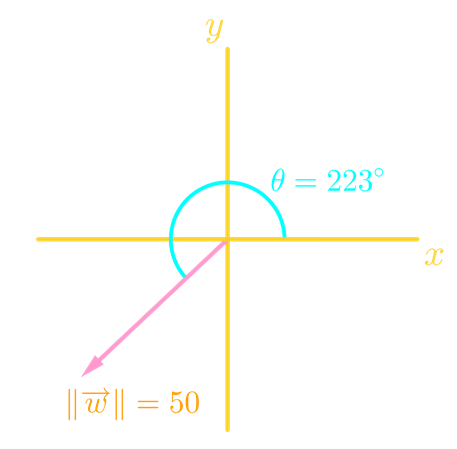• 4.
Given the magnitude and the direction angle of the following vector, determine its component form